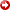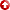--> --> Abstract: Critical Evaluation of Uncertainty Quantification Approaches for Large Scale Subsurface Problems, by Dongxiao Zhang; #90066 (2007)
[First Hit]Critical Evaluation of Uncertainty Quantification Approaches for Large Scale Subsurface Problems

Dongxiao Zhang
University of Oklahoma, Norman, OK

Subsurface flow and transport simulations are subject to uncertainties, which can result from the heterogeneity of the media and our incomplete knowledge about their properties. Such uncertainties render the model parameters random and the equations describing flow and transport in the media stochastic. Monte Carlo simulation method (MCS) is the most common approach to solve the stochastic differential equations numerically, in which a large number of realizations of the underlying field are generated and solved at high resolution. MCS is conceptually straightforward and easy to implement. However, its main disadvantage is the requirement of large computational efforts, especially for large scale problems.

Recently a number of alternative approaches have been developed to quantifying prediction uncertainties associated with subsurface modeling. This paper discusses four representative methods: The moment equation method (ME), which derives a system of deterministic differential equations governing the statistical moments (usually the first two moments) of the random variables with the method of perturbation or closure approximation; the polynomial chaos expansion method (PCE), which consists of representing the random variables using polynomial chaos basis and deriving appropriate discrete equations for the expansion coefficients using Galerkin technique; theKarhunen-Loevebased moment equation method (KLME), which decomposes the input random fields with Karhulen-Loeve (KL) expansion and the output random fields via perturbation series; and the probability collocation method (PCM), which represents the random fields with KL and polynomial chaos expansions but contrary to PCE, evaluates the expansion coefficients with a sampling (collocation) method.

The efficiency of these methods depends on how the random (probability) space is approximated. In the MCS, a number of independent realizations are used to represent (approximate) the random space, resorting to the law of large number. In the ME, the random space is assumed to be adequately approximated with the first two statistical moments (mean and covariance) so that the random dimensionality is dictated by the size of the covariance matrix. Common among the KLME, PCE and PCM approaches, the random space is approximated via a small number of principle modes in the KL expansions of the input random fields. The approximations in representing the input random fields are compared and their implications on the efficiency of the approaches are discussed. It has been found that the KLME, PCE and PCM are generally more efficient than the MCS and the ME, especially for large-scale problems. The expansions in representing the output random fields and the ways for evaluating the expansion coefficients distinguish among the KLME, PCE and PCM. Detailed theoretical analyses and numerical computations are performed to compare these three methods in terms of accuracy, efficiency, validity range, and compatibility with existing deterministic simulators.

AAPG Search and Discover Article #90066©2007 AAPG Hedberg Conference, The Hague, The Netherlands